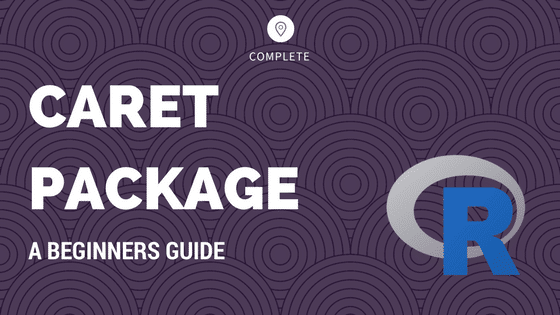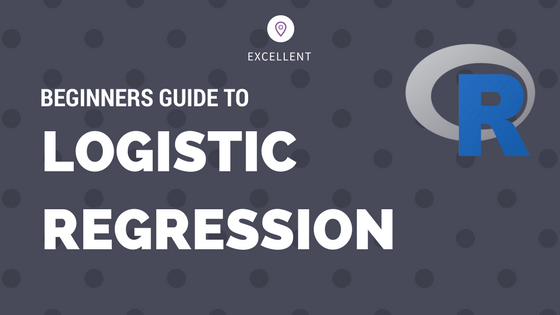Drop a Query

# Regression## Linear Regression in Julia

Linear Regression is a fundamental machine learning algorithm used to predict a numeric dependent variable based on one or more independent variables. The dependent variable (Y) should be continuous. In this tutorial I explain how to build linear regression in Julia, with full-fledged post model-building diagnostics. To know more about the concepts behind linear regression, …## Caret Package – A Practical Guide to Machine Learning in R

Caret Package is a comprehensive framework for building machine learning models in R. In this tutorial, I explain nearly all the core features of the caret package and walk you through the step-by-step process of building predictive models. Be it a decision tree or xgboost, caret helps to find the optimal model in the shortest …## Logistic Regression – A Complete Tutorial With Examples in R

Logistic regression is a predictive modelling algorithm that is used when the Y variable is binary categorical. That is, it can take only two values like 1 or 0. The goal is to determine a mathematical equation that can be used to predict the probability of event 1. Once the equation is established, it can …Course Preview

## Machine Learning A-Z™: Hands-On Python & R In Data Science

### Free Sample Videos:#### Machine Learning A-Z™: Hands-On Python & R In Data Science#### Machine Learning A-Z™: Hands-On Python & R In Data Science#### Machine Learning A-Z™: Hands-On Python & R In Data Science#### Machine Learning A-Z™: Hands-On Python & R In Data Science